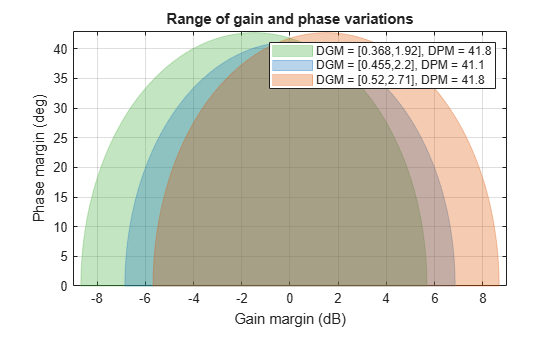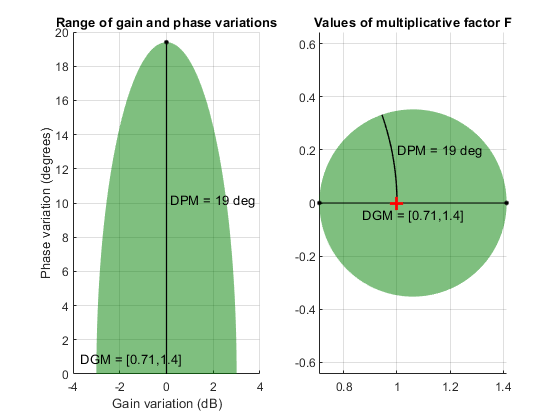# dm2gm

Get disk-based margins from disk size and skew

## Syntax

``[GM,PM] = dm2gm(alpha)``
``[DGM,DPM] = dm2gm(alpha,sigma)``

## Description

`umargin` and `diskmargin` model gain and phase variation as a multiplicative factor F(s) taking values in a disk centered on the real axis. The disk is described by two parameters: ɑ, which sets the size of the variation, and σ, or skew, which biases the gain variation toward increase or decrease. (See Algorithms for more details about this model.) The disk can alternatively be described by its real-axis intercepts `DGM = [gmin,gmax]`, which represent the relative amount of gain variation around the nominal value F = 1. Use `gm2dm` and `dm2gm` to convert between the ɑ,σ values and the disk-based gain margin `DGM = [gmin,gmax]` that describe the same disk.

example

````[GM,PM] = dm2gm(alpha)` returns the gain and phase variations modeled by the disk with disk-size `alpha` and zero skew. The disk represents a gain that can vary between `1/``GM` and `GM` times the nominal value, and a phase that can vary by ±`PM` degrees. If `alpha` is a vector, the function returns `GM` and `PM` for each entry in the vector. ```

example

````[DGM,DPM] = dm2gm(alpha,sigma)` returns the disk-based gain variation `DGM` and disk-based phase variation `DPM` corresponding to the disk parameterized by `alpha` and `sigma`. `DPM` is a vector of the form `[gmin,gmax]`, and `DPM` is a vector of the form `[-pm,pm]` corresponding to the disk size `alpha` and skew `sigma`. If `alpha` and `sigma` are vectors, then the function returns the ranges for the pairs `alpha1,sigma1;...;alphaN,sigmaN`.```

## Examples

collapse all

Determine disk-based gain and phase variations captured by a disk with size α = 0.5.

```alpha = 0.5; [GM,PM] = dm2gm(alpha)```
```GM = 1.6667 ```
```PM = 28.0725 ```

When you omit `sigma`, the `dm2gm` command returns the gain and phase variations corresponding to α with zero skew. Zero skew means that the disk represents gain that can increase or decrease by the same amount. In this case, α = 0.5 models a gain that can increase or decrease by up to a factor 1.6667 of its nominal value. The phase variation corresponding to this disk-based gain variation is ±28°. Visualize this disk.

`diskmarginplot(alpha,0,'disk')`The plot shows the values of F in complex plane corresponding to disk size `alpha` = 0.5 and `sigma` = 0. You can see that `DGM` = `[1/GM,GM]` for this disk.

Determine the disk-based gain and phase variations modeled by the disk parameterized by disk size α = 0.6 and skew σ = 0.75.

```alpha = 0.6; sigma = 0.75; [DGM,DPM] = dm2gm(alpha,sigma)```
```DGM = 1×2 0.6066 2.2632 ```
```DPM = 1×2 -34.2267 34.2267 ```

Visualize the gain and phase variations represented by this disk.

`diskmarginplot(DGM)`Because σ > 0, this disk models a gain that can increase more than it can decrease relative to the nominal value.

Determine the disk-based gain and phase variations represented by disks of the same size but with different skews.

```alpha = 0.75; sigma = [-0.5;0;0.5]; [DGM,DPM] = dm2gm(alpha,sigma)```
```DGM = 3×2 0.3684 1.9231 0.4545 2.2000 0.5200 2.7143 ```
```DPM = 3×2 -41.7908 41.7908 -41.1121 41.1121 -41.7908 41.7908 ```

The disks capture roughly similar phase variations, but the skew biases the disk toward gain decrease or increase. For the disk with zero skew, the gain variation is balanced, and meaning that gain can increase or decrease by the same amount. To Visualize the simultaneous range of gain and phase variations corresponding to each row in `DGM`.

`diskmarginplot(DGM)`## Input Arguments

collapse all

Disk size, specified as a scalar or vector. Disk-based gain-margin analysis represents gain and phase variation as a multiplicative uncertainty F, which is a disk of values containing F = 1, corresponding to the nominal value of the system. The disk is parameterized by `alpha`, which sets the size of the disk, and `sigma`, which biases the gain variation toward gain increase or decrease. See Algorithms for details about the meaning of `alpha`.

To obtain gain and phase variations corresponding to multiple disk sizes, specify `alpha` as a vector.

Skew, specified as a scalar or vector. The skew biases the modeled gain variation toward gain increase or decrease.

• `sigma` = 0 for a balanced gain range `[gmin,gmax]`, with `gmin = 1/gmax`.

• `sigma` is positive for a varying gain that can increase more than it can decrease, `gmax > 1/gmin`.

• `sigma` is negative for a varying gain that can decrease more than it can increase, `gmin < 1/gmax`.

The more the gain range is biased, the larger the absolute value of `sigma`. For additional details about the meaning of `sigma`, see Algorithms.

To obtain gain and phase variations corresponding to multiple disks of varying skew, specify `sigma` as a vector.

## Output Arguments

collapse all

Amount of gain increase or decrease in absolute units, returned as a real scalar or a vector.

• If `alpha` is a real scalar and you omit `sigma`, then `dm2gm` returns a scalar `GM` such that the disk of size `alpha` models a symmetric gain variation in the range `[1/GM,GM]` and the corresponding phase variation, `[-PM,PM]`. For instance, `GM` = 2 means that the disk models a gain that can increase or decrease by a factor of 2.

• If `alpha` is a vector of form `[alpha1;...;alphaN]` and you omit `sigma`, the function returns `GM` as a column vector of the corresponding amounts of gain increase or decrease.

Amount of phase variation in degrees, returned as a real scalar or a vector.

• If `alpha` is a real scalar and you omit `sigma`, then `dm2gm` returns a scalar `PM` such that the disk of size `alpha` models a symmetric gain variation in the range `[1/GM,GM]` and the corresponding phase variation, `[-PM,PM]`. For instance, `PM` = 20 means that the disk models a phase that can increase or decrease by 20°.

• If `alpha` is a vector of form `[alpha1;...;alphaN]` and you omit `sigma`, the function returns `PM` as a column vector of the corresponding amounts of phase variation.

Range of relative gain variation, returned as a two-element vector of the form `[gmin,gmax]`, where `gmin` < 1 and `gmax` > 1. For instance, `DGM = [0.8 1.5]` represents a gain that can vary between 80% and 150% of its nominal value (that is, change by a factor between 0.8 and 1.5). `DGM` is the gain variation modeled by the disk parameterized by the input arguments `alpha` and `sigma`. It is the range in which the disk crosses the real axis. `gmin` can be negative for large negative values of `sigma`, defining a range of relative gain variation that includes a change in sign. For more information about the disk-based uncertainty model, see Algorithms.

You can use `DGM` to create a `umargin` object that represents the gain and phase uncertainty described by the disk. You can visualize the disk and the associated gain and phase variations using `diskmarginplot`.

If `alpha` and `sigma` are vectors, then `DGM` is a two-column matrix of the form ```[gmin1,gmax1; ...;gminN,gmaxN]```, where each row is the disk-based gain range corresponding to `[alpha1,sigma1; ...;alphaN,sigmaN]`.

Disk-based phase variation, returned as a two-element vector or a two-column matrix.

The vector `DPM = [-pm,pm]`, represents the relative phase variation amount determined by the geometry of the disk described by `alpha` and `sigma`. For more information, see Algorithms.

If `alpha` and `sigma` are vectors, then `DPM` is a two-column matrix of the form ```[-pm1,pm1; ...;-pmN,pmN]```, where each row is the phase variation corresponding to `[alpha1,sigma1; ...;alphaN,sigmaN]`.

## Algorithms

`umargin` and `diskmargin` model gain and phase variations in an individual feedback channel as a frequency-dependent multiplicative factor F(s) multiplying the nominal open-loop response L(s), such that the perturbed response is L(s)F(s). The factor F(s) is parameterized by:

`$F\left(s\right)=\frac{1+\alpha \left[\left(1-\sigma \right)/2\right]\delta \left(s\right)}{1-\alpha \left[\left(1+\sigma \right)/2\right]\delta \left(s\right)}.$`

In this model,

• δ(s) is a gain-bounded dynamic uncertainty, normalized so that it always varies within the unit disk (||δ|| < 1).

• ɑ sets the amount of gain and phase variation modeled by F. For fixed σ, the parameter ɑ controls the size of the disk. For ɑ = 0, the multiplicative factor is 1, corresponding to the nominal L.

• σ, called the skew, biases the modeled uncertainty toward gain increase or gain decrease.

The factor F takes values in a disk centered on the real axis and containing the nominal value F = 1. The disk is characterized by its intercept `DGM = [gmin,gmax]` with the real axis. `gmin` < 1 and `gmin` > 1 are the minimum and maximum relative changes in gain modeled by F, at nominal phase. The phase uncertainty modeled by F is the range `DPM = [-pm,pm]` of phase values at the nominal gain (|F| = 1). For instance, in the following plot, the right side shows the disk F that intersects the real axis in the interval [0.71,1.4]. The left side shows that this disk models a gain variation of ±3 dB and a phase variation of ±19°.

```DGM = [0.71,1.4] F = umargin('F',DGM) plot(F)````gm2dm` and `gm2dm` converts between these two ways of specifying a disk of multiplicative gain and phase uncertainty: a gain-variation range of the form `DGM = [gmin,gmax]`, and the ɑ,σ parameterization of the corresponding disk.

For further details about the uncertainty model for gain and phase variations, see Stability Analysis Using Disk Margins.

## Version History

Introduced in R2020a